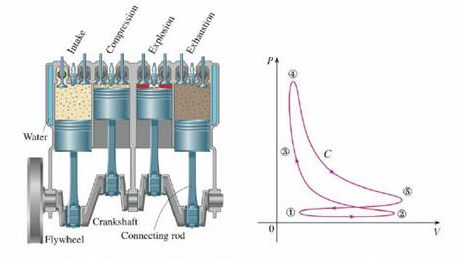Chapter 16, Problem 6P

Chapter
Section
Textbook Problem

The figure depicts the sequence of events in each cylinder of a four-cylinder internal combustion engine. Each piston moves up and down and is connected by a pivoted arm to a rotating crankshaft. Let P(t) and V(t) be the pressure and volume within a cylinder at time t, where a ≤ t ≤ b gives the time required for a complete cycle. The graph shows how P and V vary through one cycle of a four-stroke engine.During the intake stroke (from ① to ②) a mixture of air and gasoline at atmospheric pressure is drawn into a cylinder through the intake valve as the piston moves downward. Then the piston rapidly compresses the mix with the valves closed in the compression stroke (from ② to ③) during which the pressure rises and the volume decreases. At ③ the sparkplug ignites the fuel, raising the temperature and pressure at almost constant volume to ④. Then, with valves closed, the rapid expansion forces the piston downward during the power stroke (from ④ to ⑤). The exhaust valve opens, temperature and pressure drop, and mechanical energy stored in a rotating flywheel pushes the piston upward, forcing the waste products out of the exhaust valve in the exhaust stroke. The exhaust valve closes and the intake valve opens. We’re now back at ① and the cycle starts again.(a) Show that the work done on the piston during one cycle of a four-stroke engine is W = ∫C P dV, where C is the curve in the PV-plane shown in the figure. [Hint: Let x(t) be the distance from the piston to the top of the cylinder and note that the force on the piston is F = AP(t) i, where A is the area of the top of the piston. Then W = ∫ C 1 F · dr, where C1 is given by r(t)= x(t) i, a ≤ t ≤ b. An alternative approach is to work directly with Riemann sums.](b) Use Formula 16.4.5 to show that the work is the difference of the areas enclosed by the two loops of C.

(a)

To determine

To show: The work done on the piston during one cycle of a four-stroke engine is W=CPdV .

Explanation

Formula used:

Write the expression for work done as follows.

W=CFdr (1)

Consider the top of the cylinder is at the origin and the distance from the top of the cylinder to the piston at time t is x(t)0 . Consider the curve C1 be the curve traced by the piston during one four-stroke cycle is represented as follows.

r(t)=x(t)i atb

Differentiate r(t) with respect to t .

dr(t)=x(t)idt

Consider A is the area of the piston and P(t)i is the pressure applied on the area.

Write the expression for force on the piston as follows.

F=AP(t)i

Modify equation (1) as follows.

W=C1Fdr(t)

Substitute AP(t)i for F and x(t)idt for dr(t) ,

W=C1AP(t)ix(t)idt

Apply the limit for integration as follows

(b)

To determine

To show: The work is the difference of the areas enclosed by the two loops of C.

Still sussing out bartleby?

Check out a sample textbook solution.

See a sample solution

The Solution to Your Study Problems

Bartleby provides explanations to thousands of textbook problems written by our experts, many with advanced degrees!

Get Started

Find all possible real solutions of each equation in Exercises 3144. y32y22y3=0

Finite Mathematics and Applied Calculus (MindTap Course List)

Prove the statement using the , definition of a limit. limx2(x21)=3

Single Variable Calculus: Early Transcendentals, Volume I

Using Geometry Use a geometric argument to show that 0309y29x2y2dxdy=92.

Calculus: Early Transcendental Functions (MindTap Course List)

The radius of convergence of is: 1 3 ∞

Study Guide for Stewart's Single Variable Calculus: Early Transcendentals, 8th